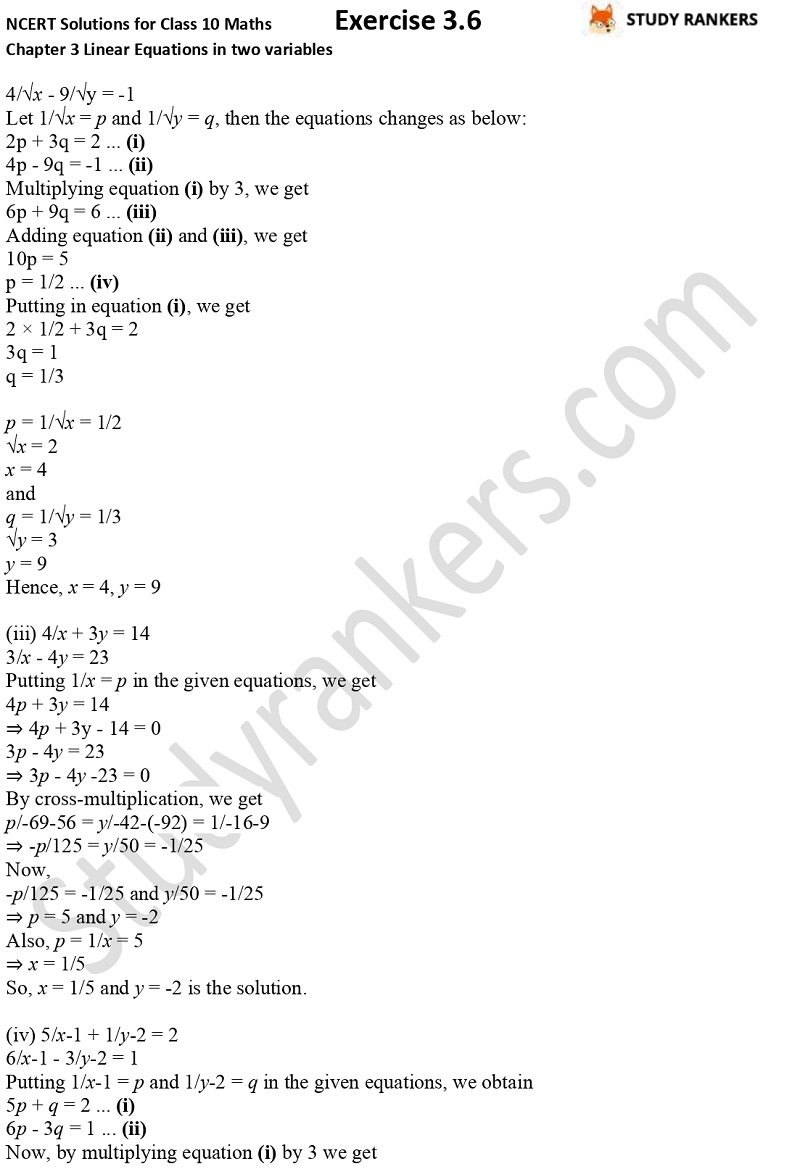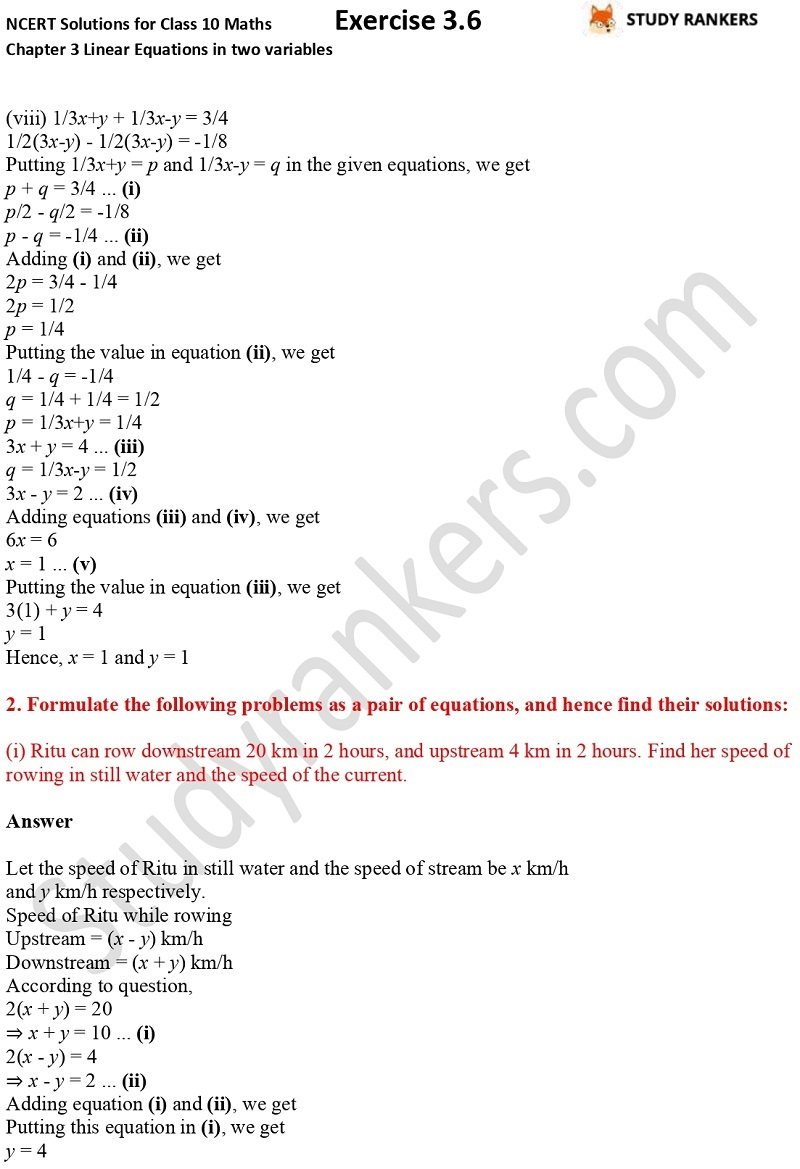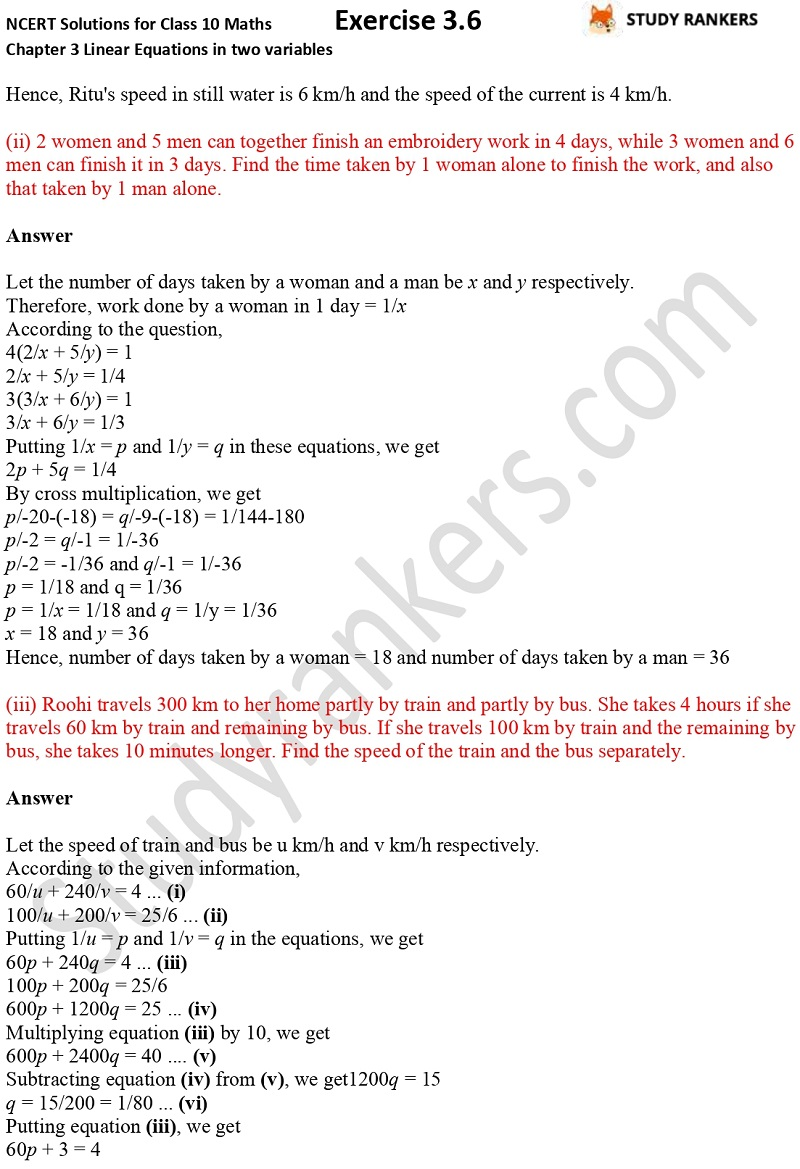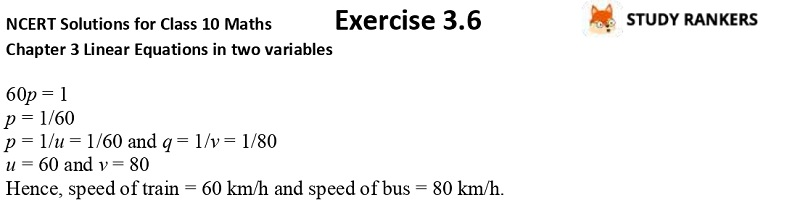>

## NCERT Solutions for Class 10 Maths Chapter 3 Pair of Linear Equations in Two Variables Exercise 3.6

NCERT Solutions for Class 10 is the best to cover up the basic of the subject. Here, you will find Chapter 3 Pair of Linear Equations in two variables Maths Class 10 NCERT Solutions that will help you in improving your performance and knowledge of the chapter. The concepts in the exercise will also help you when you go for higher classes. These Chapter 3 Class 10 Maths solutions are prepared by Studrankers experts which will clear your difficult doubts. There are only two questions in this exercise in which you have to solve the given pair of linear equations.X